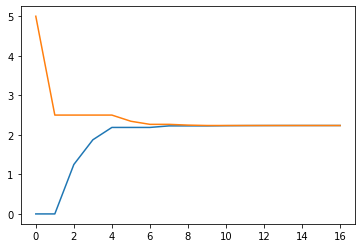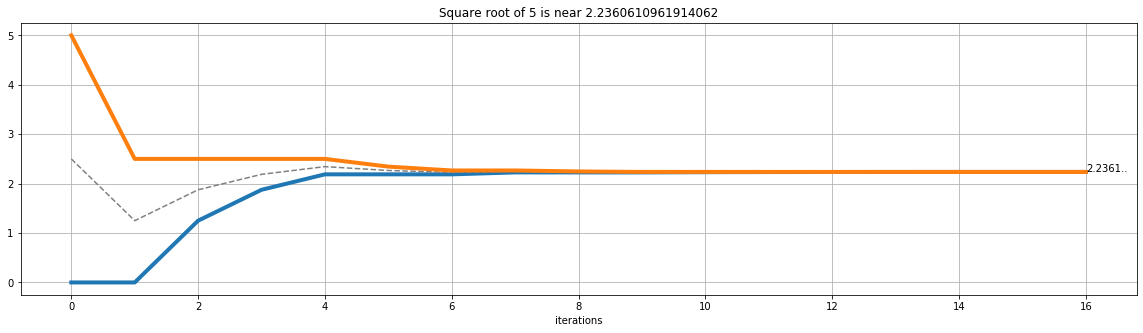# Bisection Search

In mathematics, the bisection algorithm is a simple method for approximating the roots of one-dimentional functions. It is also known as the interval halving method or the binary search method.

In this case, the function is the square root of a positive real number.

Since for many numbers (e.g. 2), the solution is not a rational number, there is no way to precisely represent it as a finite string of digits. We will look for an approximation.

In this case, the bisection search algorithm start with a range that is known to contain the solution. For example, if we want to find the square root of 5, we know that the range (0,5) contains the square root of 5.

We arbitrarily try with the midpoint, in this case 2.5, and check how far 2.5^2 is from the solution.

$$2,5^2 = 6.5 > 5$$

Okay, that was not a good approximation, since we have an error of 1.5.

Now, after one step, we know that the solution lies between 0 and 2.5. Our search space is divided in half. We repeat the previous step:

1. Find the midpoint c
2. Calculate the function value at the midpoint$$f( c) = c^2$$
3. Check if the error is sufficiently small (satisfactory) or not: $$f( c) - a$$ where a is the value whose square root we are trying to approximate.
4. If the error is sufficiently small, return c, otherwise, iterate again from step 1.

Below, we implement this method in Python.

References:

import numpy as np
import matplotlib.pyplot as plt

def square_root(target: float, max_error=0.0001):
""" Function for finding the square root of a positive number,
returns an approximation of the square root of the target
"""
target = abs(target)  # for negative inputs
low: float = 0  # low and high define the search interval
high: float = target
# find the midpoint of the search interval.
attempt: float = (low + high) / 2
# check if the approximation is good enough.
while abs(attempt ** 2 - target) > max_error:
# if the approximation is too high, use the
# midpoint as upper limit for the new search interval.
if attempt ** 2 > target:
high = attempt
# if the approximation is too low,
# use the midpoint as new lower limit for the search interval.
else:
low = attempt
attempt = (low + high) / 2
return attempt  # if the approximation is good enough, return it

# sanity check
print(square_root(5))
print(square_root(5) ** 2)

2.2360610961914062
4.999969225900713

X = 5
FFLOW = 0
FFHIGH = X
LAMBDA = 0.0001
ATTEMPT = (FFLOW + FFHIGH) / 2
# print(ATTEMPT**2 - X)
iteration = 0
ITERATIONS =   # TODO: convert to numpy
FFLOWS = [FFLOW]  # TODO: convert to numpy
FFHIGHS = [FFHIGH]  # TODO: convert to numpy
while abs(ATTEMPT ** 2 - X) > LAMBDA:
# print(f'error ={ATTEMPT**2 - X}')
if ATTEMPT ** 2 > X:
FFHIGH = ATTEMPT
else:
FFLOW = ATTEMPT
FFLOWS.append(FFLOW)
FFHIGHS.append(FFHIGH)
iteration += 1
ITERATIONS.append(iteration)
ATTEMPT = (FFLOW + FFHIGH) / 2
print(ATTEMPT)
print(ATTEMPT ** 2)
print(f"iterations {ITERATIONS}")
print(FFLOWS)
print(FFHIGHS)
fig, ax = plt.subplots()
plt.plot(ITERATIONS, FFLOWS)
plt.plot(ITERATIONS, FFHIGHS)
plt.show()

2.2360610961914062
4.999969225900713
iterations [0, 1, 2, 3, 4, 5, 6, 7, 8, 9, 10, 11, 12, 13, 14, 15, 16]
[0, 0, 1.25, 1.875, 2.1875, 2.1875, 2.1875, 2.2265625, 2.2265625, 2.2265625, 2.2314453125, 2.23388671875, 2.235107421875, 2.2357177734375, 2.23602294921875, 2.23602294921875, 2.23602294921875]
[5, 2.5, 2.5, 2.5, 2.5, 2.34375, 2.265625, 2.265625, 2.24609375, 2.236328125, 2.236328125, 2.236328125, 2.236328125, 2.236328125, 2.236328125, 2.236175537109375, 2.2360992431640625]def square_root_plot(target: float, max_error=0.0001, ax=ax):
""" Function for finding the square root of a positive number, returns an approximation of the square root of the target
and a plot that shows how the search range converges. """
target: abs(target)  # for negative targets
low: float = 0  # low and high define the search interval
high: float = target
attempt: float = (low + high) / 2  # find the midpoint of the search interval.
iteration = 0
iterations: list = np.array()
lows: np.array = np.array([low])
attempts: np.array = np.array([attempt])
highs: np.array = np.array([high])
while (
abs(attempt ** 2 - target) > max_error
):  # check if the approximation is good enough.
if (
attempt ** 2 > target
):  # if the approximation is too high, use the midpoint as upper limit for the new search interval.
high = attempt
else:  # if the approximation is too low, use the midpoint as new lower limit for the search interval.
low = attempt
iteration += 1
attempt = (low + high) / 2
iterations = np.append(iterations, iteration)
lows = np.append(lows, low)
attempts = np.append(attempts, attempt)
highs = np.append(highs, high)
# return (attempt,fig) # if the approximation is good enough, return it
(line,) = ax.plot(
iterations, lows, linewidth=4
)  # add a line showing the search interval's lower bound per iteration
(line,) = ax.plot(
iterations, attempts, color="grey", linestyle="dashed"
)  # add a line showing the midpoints per iteration
(line,) = ax.plot(
iterations, highs, linewidth=4
)  # add a line showing the search interval's upper bound per iteration
plt.text(iteration, attempt, f"{attempt:.4f}..")
ax.grid()  # Turn on the grid
ax.set_title(f"Square root of {target} is near {attempt}")
ax.set_xlabel("iterations")
return line

fig, ax = plt.subplots(figsize=(20, 5))
square_root_plot(5, ax=ax)
plt.show()fig, ax = plt.subplots(figsize=(20, 5))
square_root_plot(5, ax=ax)
plt.show()fig, (ax1, ax2, ax3) = plt.subplots(figsize=(20, 20), nrows=3)
square_root_plot(2, ax=ax1)
square_root_plot(5, ax=ax2)
square_root_plot(15, ax=ax3)
plt.show()Using a minor modification, we can change the square_root function to find cube roots:

def cube_root(target: float, max_error=0.0001):
""" Function for finding the square root of a positive number,
returns an approximation of the square root of the target.
target cannot be negative
"""
if target < 0:
raise ValueError("Only greater than zero are allowed")
low: float = 0  # low and high define the search interval
high: float = target
# find the midpoint of the search interval.
attempt: float = (low + high) / 2
# check if the approximation is good enough.
while abs(attempt ** 3 - target) > max_error:
# if the approximation is too high, use the
# midpoint as upper limit for the new search interval.
if attempt ** 3 > target:
high = attempt
# to debug
# print(f"high: {high} - error: {attempt ** 3 - target}")
# if the approximation is too low,
# use the midpoint as new lower limit for the search interval.
else:
low = attempt
# to debug
# print(f"low: {low} - error: {attempt ** 3 - target}")
attempt = (low + high) / 2
return attempt  # if the approximation is good enough, return it

cube_root(125)

4.999998956918716


And we can easily create a general function for finding square roots given positive bases and inputs

def general_root(target: float, base: float, max_error: float = 0.0001):
""" Function for finding the square root of a positive number,
returns an approximation of the square root of the target.
target cannot be negative
"""
if target < 0:
raise ValueError("positive or zero are allowed")
if base <= 0:
raise ValueError("only bases greater than zero are allowed")
low: float = 0  # low and high define the search interval
high: float = target
# find the midpoint of the search interval.
attempt: float = (low + high) / 2
# check if the approximation is good enough.
while abs(attempt ** base - target) > max_error:
# if the approximation is too high, use the
# midpoint as upper limit for the new search interval.
if attempt ** base > target:
high = attempt
# to debug
# print(f"high: {high} - error: {attempt ** 3 - target}")
# if the approximation is too low,
# use the midpoint as new lower limit for the search interval.
else:
low = attempt
# to debug
# print(f"low: {low} - error: {attempt ** 3 - target}")
attempt = (low + high) / 2
return attempt  # if the approximation is good enough, return it


$\sqrt{4}$

general_root(4, 2)

2.0


$\sqrt{8}$

general_root(8, 3)

2.0


$\sqrt{16}$

general_root(16, 4)

2.0

%%time
general_root(4, 1)

CPU times: user 16 µs, sys: 1 µs, total: 17 µs
Wall time: 21.5 µs

3.99993896484375

%%time
general_root(2, 2)

CPU times: user 16 µs, sys: 1 µs, total: 17 µs
Wall time: 21 µs

1.4141845703125


The purpose of this notebook was not to get a production ready root finding function, there ary many complex math algorithms for doing this. But we can perform some simple optimizations, for example if the base is 1, return the target without doing further optimizations.

Further, we could also hardcode some commonly used roots, like the square root of 1, 2, 3, 4, and so on. Below, only the square root of 2 was hardcoded.

def general_root(target: float, base: float, max_error: float = 0.0001):
""" Function for finding the square root of a positive number,
returns an approximation of the square root of the target.
target cannot be negative
"""
if target < 0:
raise ValueError("positive or zero are allowed")
if base <= 0:
raise ValueError("only bases greater than zero are allowed")
if base == 1:
return target
if target == 2 and base == 2:
return 1.41421356237
low: float = 0  # low and high define the search interval
high: float = target
# find the midpoint of the search interval.
attempt: float = (low + high) / 2
# check if the approximation is good enough.
while abs(attempt ** base - target) > max_error:
# if the approximation is too high, use the
# midpoint as upper limit for the new search interval.
if attempt ** base > target:
high = attempt
# to debug
# print(f"high: {high} - error: {attempt ** 3 - target}")
# if the approximation is too low,
# use the midpoint as new lower limit for the search interval.
else:
low = attempt
# to debug
# print(f"low: {low} - error: {attempt ** 3 - target}")
attempt = (low + high) / 2
return attempt  # if the approximation is good enough, return it

%%time
general_root(4, 1)

CPU times: user 5 µs, sys: 0 ns, total: 5 µs
Wall time: 9.06 µs

4

%%time
general_root(2, 2)

CPU times: user 5 µs, sys: 0 ns, total: 5 µs
Wall time: 9.78 µs

1.41421356237

Previous